# Exact Cover Problem and Algorithm X | Set 1

• Difficulty Level : Expert
• Last Updated : 15 Feb, 2018

If you have ever tried to create a program for solving Sudoku, you might have come across the Exact Cover problem. In this article, we will discuss what is the exact cover problem and an algorithm “Algorithm X” proposed by Donald Knuth to solve this problem.

Given a collection S of subsets of set X, an exact cover is the subset S* of S such that each element of X is contained is exactly one subset of S*. It should satisfy following two conditions –

• The Intersection of any two subsets in S* should be empty. That is, each element of X should be contained in at most one subset of S*
• Union of all subsets in S* is X. That means union should contain all the elements in set X. So we can say that S* covers X.

Example (standard representation) –
Let S = { A, B, C, D, E, F } and X = {1, 2, 3, 4, 5, 6, 7} such that –

• A = {1, 4, 7}
• B = {1, 4}
• C = {4, 5, 7}
• D = {3, 5, 6}
• E = {2, 3, 6 7}
• F = {2, 7}

Then S* = {B, D, F} is an exact cover, because each element in X is contained exactly once in subsets {B, D, F} . If we union subsets then we will get all the elements of X –
[Tex]B \bigcup D \bigcup F = \{ 1,2,3,4,5,6,7\}[\Tex]

The Exact cover problem is a decision problem to determine if exact cover exists or not. It is considered to be NP-Complete problem.

The problem can be represented in the form of a matrix where the row represents the subsets of S and columns represent the element of X. The above problem can be represented as –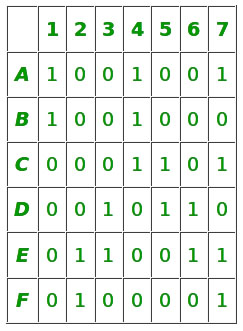problem matrix

In the context of matrix representation, our exact cover is the selection of rows such that each column contains only single 1 among selected rows. So we can see below that each column have only single 1 among selected rows B, D, F.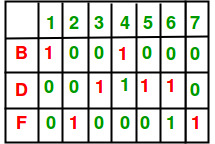exact cover

Algorithm X

Donald Knuth proposed an Algorithm X which can find all the solutions to the exact cover problem. Algorithm X can be efficiently implemented by “dancing links” technique proposed by Donald Knuth called DLX.

Algorithm X is recursive, depth-first, backtracking algorithm. It is non-deterministic in nature, that means for the same input, it can exhibit different behaviors on a different run.
Following is the pseudo code for Algorithm X –

```
1. If the matrix A has no columns, the current partial solution
is a valid solution; terminate successfully.
2. Otherwise, choose a column c (deterministically).
3. Choose a row r such that A[r] = 1 (nondeterministically).
4. Include row r in the partial solution.
5. For each column j such that A[r][j] = 1,
for each row i such that A[i][j] = 1,
delete row i from matrix A.
delete column j from matrix A.
6. Repeat this algorithm recursively on the reduced matrix A.
```

Non-deterministic choice of r means, algorithm copy itself into sub algorithm. Each sub algorithm inherit original matrix A but reduces it with respect to chosen r (we will see this shortly in example)

The sub algorithm forms a search tree with the original problem at the root and each level k have sub algorithm correspond to the rows chosen in previous level (just like the n-queen search space).

If chosen column c is entirely zero then there are no sub algorithms and the process terminated unsuccessfully. Knuth suggests that we should choose the column with the minimum number of 1’s in it. If no column left, then we know we have found our solution.

Example
Consider the above example, we will apply Algorithm X on it to find the exact cover –

Level – 0
Step-1: Our matrix is not empty, it has columns then proceed
Step-2: The first column which has minimum number of 1’s in it is C-1 so we will select it
Step-3: Rows A and B have 1 at C-1 so they are selected.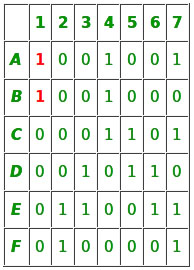So now the algorithm moves to first branch at level 1

Level – 1 (Select row A)
Step-4: Select row A and add it to partial solutions
Step-5: Row A has 1 in column 1, 4, 7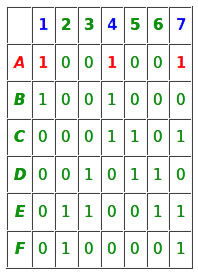C-1 have 1 in row A and B, C-4 have 1 in A, B and C, C-7 have 1 in row A, C, E, and F.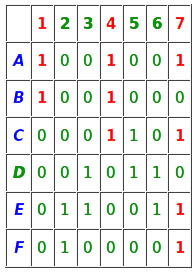So column 1, 4, 7 and rows A, B, C, E and F should be removed.Step 1 – Matrix is not empty so proceed
Step 2 – The first column which has minimum number of 1’s is C-2
Since column C-2 have no 1’s in it, our search will terminate here unsuccessfully.
Now our algorithm will backtrack at level 0 and proceed with row B at second branch at level 1

Level – 1(Select row B)
Step – 4: Select row B and add it to partial solutions
Step – 5: Row B has 1 in column C-1 and C-4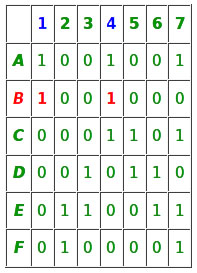C-1 have 1’s at row A and B. C-4 have 1’s in row A, B and C.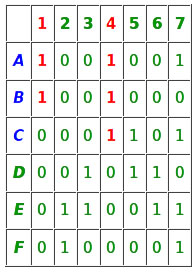So C-1, C-2 and Row A, B, C will be removed from the matrix.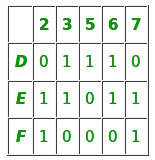Now we repeat algorithm –
Step-1: Matrix is not empty, proceed
Step-2: C-5 has a minimum number of 1’s in it, so it is chosen.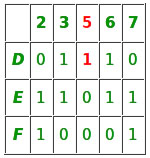Step-3: Row D has 1 at C-5, so it is chosen
Now algorithm moves to 1st branch at level 2 with matrix having row D, E and F

Level-2(Select row D)
Step-4: Row D is chosen and added to partial solution.
Step-5: C-3, C-5, C-6 have 1 at row D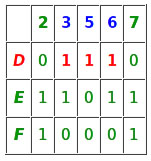At C-3 row D and E have 1, at C-5 row D have 1 and at C-6 row D, E have 1.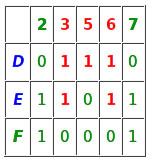So these rows and columns should be deleted and we left with a matrix having only row F and column 2, 7.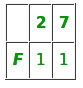Now we will repeat the algorithm –
Step-1: Matrix is not empty so proceed
Step-2: C-2 is the first column having a minimum number if 1’s in it. So it is chosen
Step-3: Row F have 1 at C-2 so it is chosen.
Now algorithm will move to the first branch at level 3.

Level – 3(Select row F)
Step-4: Row F is added to the partial solution
Step-5: C-2 and C-7 have 1 at row F.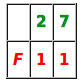C-2 have 1 at row F, C-7 have 1 at row F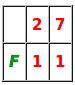So C2,C7 and row F should be removed. After removing we will left with an empty matrix so our search can terminate here successfully and we have our exact cover {B, D,F}

sub algorithm backtrack at level 2 and since there is no row left at level 3.
It further backtracks at level 1 . Since at level 1 there is no row left to our algorithm terminated.

In next article, we will discuss how to implement DLX efficiently to solve Exact Cover.

References

This article is contributed by Atul Kumar. If you like GeeksforGeeks and would like to contribute, you can also write an article using contribute.geeksforgeeks.org or mail your article to contribute@geeksforgeeks.org. See your article appearing on the GeeksforGeeks main page and help other Geeks.